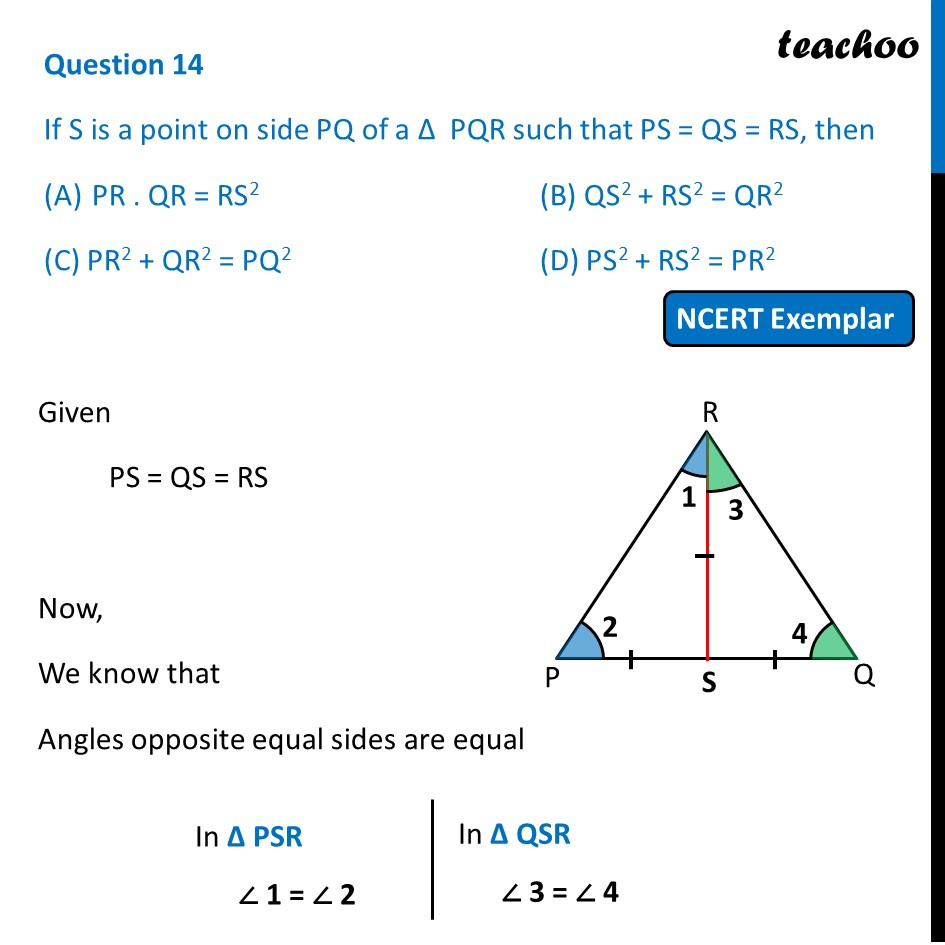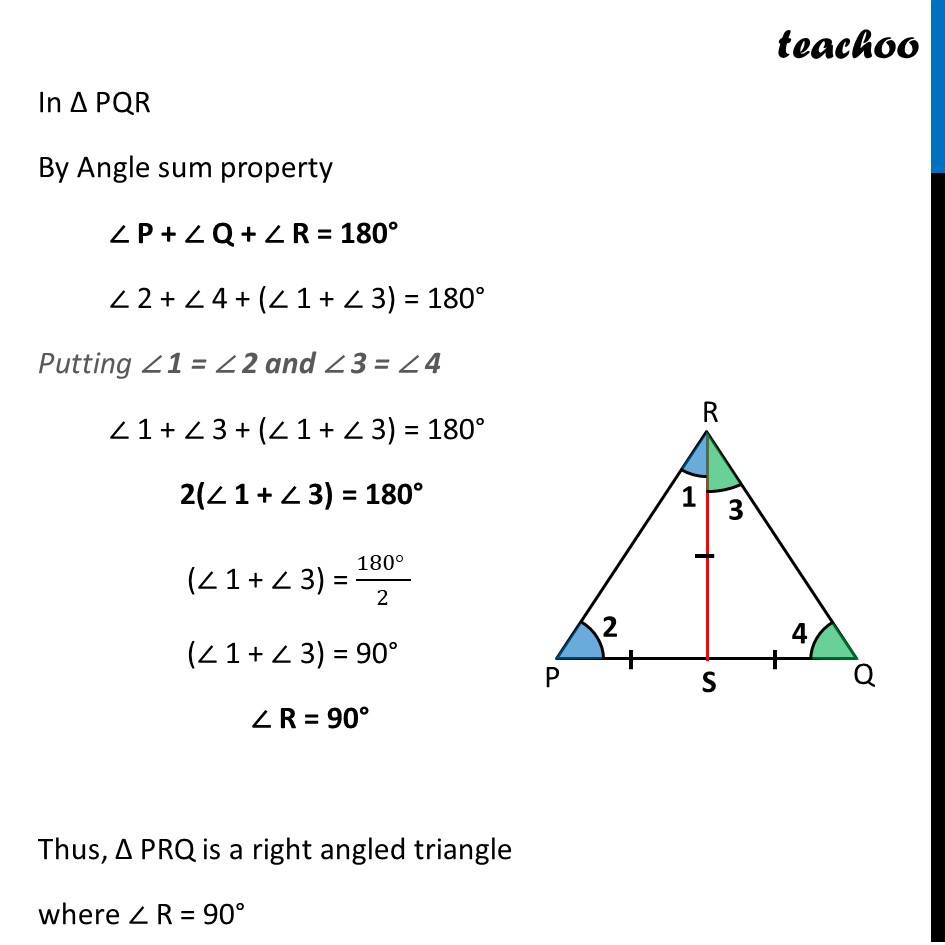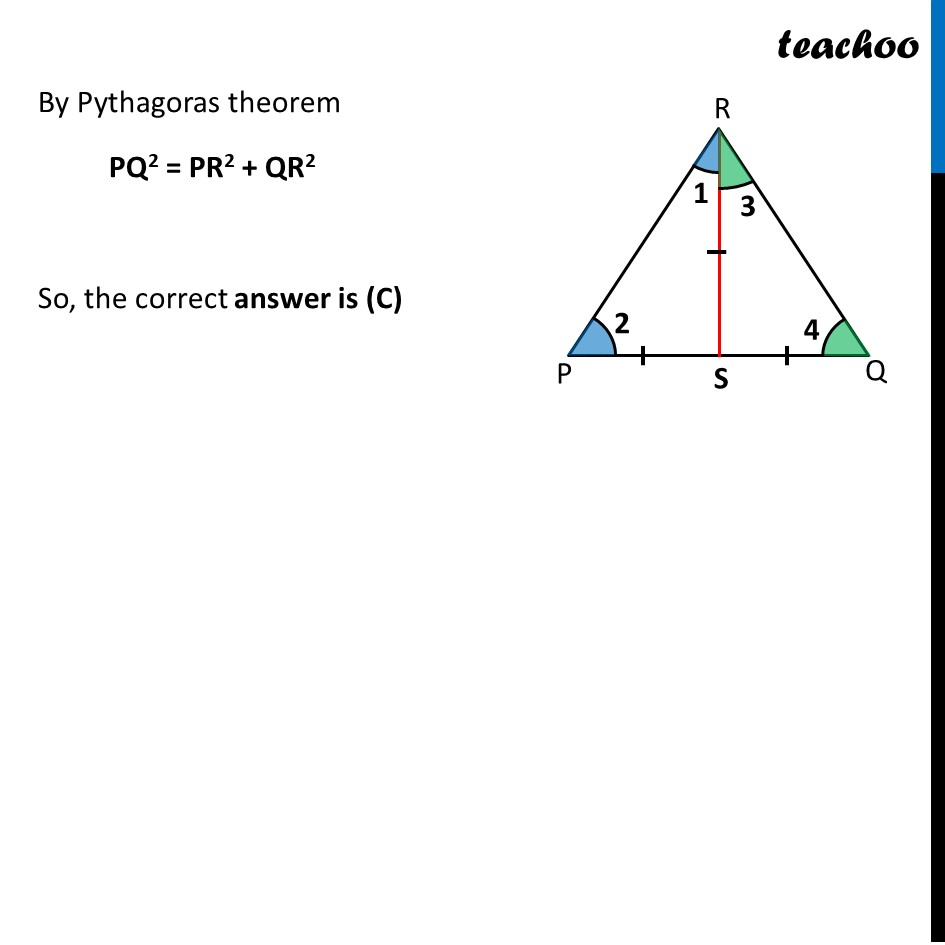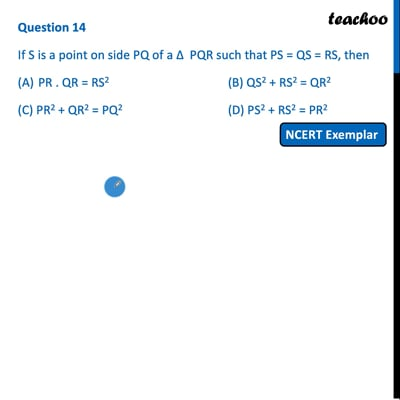NCERT Exemplar - MCQ

Chapter 6 Class 10 Triangles
Serial order wise

## (D) PS 2 + RS 2 = PR 2This video is only available for Teachoo black users

Introducing your new favourite teacher - Teachoo Black, at only ₹83 per month

### Transcript

Question 14 If S is a point on side PQ of a ∆ PQR such that PS = QS = RS, then PR . QR = RS2 (B) QS2 + RS2 = QR2 (C) PR2 + QR2 = PQ2 (D) PS2 + RS2 = PR2 Given PS = QS = RS Now, We know that Angles opposite equal sides are equal In Δ PSR ∠ 1 = ∠ 2 In Δ QSR ∠ 3 = ∠ 4 In Δ PQR By Angle sum property ∠ P + ∠ Q + ∠ R = 180° ∠ 2 + ∠ 4 + (∠ 1 + ∠ 3) = 180° Putting ∠ 1 = ∠ 2 and ∠ 3 = ∠ 4 ∠ 1 + ∠ 3 + (∠ 1 + ∠ 3) = 180° 2(∠ 1 + ∠ 3) = 180° (∠ 1 + ∠ 3) = (180° )/2 (∠ 1 + ∠ 3) = 90° ∠ R = 90° Thus, Δ PRQ is a right angled triangle where ∠ R = 90° By Pythagoras theorem PQ2 = PR2 + QR2 So, the correct answer is (C)